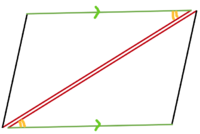# Help needed on problem

#### Hannah1234

##### New member
I’m confused is anyone able to help

#### Attachments

• 734.4 KB Views: 18

#### Davey Jones

##### New member
Well, your two triangles already have two pairs of congruent sides (AC and SE as stated, and the shared side CS). You have a couple of theorems for triangle similarity involving two sides: SSS, and SAS. So, you need to either show sides AS and CE are congruent (giving SSS), or the included angles are congruent between the sides we already know about (so angles ACS and ESC, thus giving SAS).

So, which would be easier to show congruent: the third sides, or the included angles?

hint: I haven’t yet taken advantage of the fact that AC and SE are parallel, or the fact that CS is a transversal to the parallel segments.

#### skeeter

##### Elite Member
looks like you can use SAS to prove the two triangles are congruent

#### pka

##### Elite Member
I’m confused is anyone able to help
.
You cannot prove the two triangles equal they may be congruent Is that what you mean?

#### Hannah1234

##### New member
Well, your two triangles already have two pairs of congruent sides (AC and SE as stated, and the shared side CS). You have a couple of theorems for triangle similarity involving two sides: SSS, and SAS. So, you need to either show sides AS and CE are congruent (giving SSS), or the included angles are congruent between the sides we already know about (so angles ACS and ESC, thus giving SAS).

So, which would be easier to show congruent: the third sides, or the included angles?

hint: I haven’t yet taken advantage of the fact that AC and SE are parallel, or the fact that CS is a transversal to the parallel segments.
Any way you can break it down a little more

#### Subhotosh Khan

##### Super Moderator
Staff member
Any way you can break it down a little more
Well, your two triangles already have two pairs of congruent sides (AC and SE as stated, and the shared side CS). You have a couple of theorems for triangle similarity involving two sides: SSS, and SAS. So, you need to either show sides AS and CE are congruent (giving SSS), or the included angles are congruent between the sides we already know about (so angles ACS and ESC, thus giving SAS).

So, which would be easier to show congruent: the third sides, or the included angles?

hint: I haven’t yet taken advantage of the fact that AC and SE are parallel, or the fact that CS is a transversal to the parallel segments.
Exactly where are you getting lost?

You have a couple of theorems for triangle similarity involving two sides: SSS, and SAS

If you don't know those theorems of triangle congruency (Side-side-side and Angle-side-angle) look up in your text-book or use Google.

Can you post your work/understanding prior to get lost?

#### Hannah1234

##### New member
Over all I’m getting lost I don’t get this at all

#### lev888

##### Senior Member
Over all I’m getting lost I don’t get this at all
We can't help you if you don't contribute to the process. If you have been asked to prove that 2 triangles are congruent you must have been studying some methods of doing it. What are they?

#### lex

##### Full MemberTop and bottom lines are parallel, so the marked angles are equal ('alternate angles are equal').
The coloured lines are known to be equal (red/red and green/green).
So each triangle has a green side, red side and a yellow angle in-between.
Showing this is sufficient to say that the two triangles are congruent.
That is what we mean by "SAS" - it is a theorem that when two sides and the angle in-between are the same in both triangles, then the two triangles are known to be congruent.